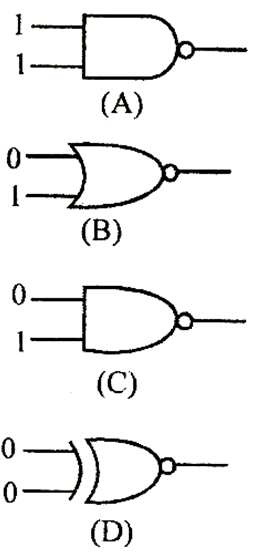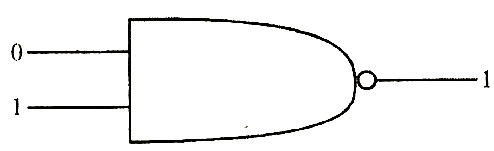# Which of the following gates will have an output of I?(a)    D        (b)    A        (c)    B        (d)    CAditi Chauhan
9 years ago

(d)

(a)    is a NAND gate so output is ¯(1 ×1)  =¯1= 0
(b)    is a NOR gate so output is ¯(0 + 1)  =¯1  =0
(c)    is a NAND gate so output is ¯(0 × 1)  = ¯0  = 1
(d)    is a XOR gate so output is 0?0 = 0Following is NAND gate
Y=¯(A.B)